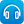# Mathematics / Year 7 / Number and Algebra / Number and place value

Version 8
Curriculum content descriptions

Investigate and use square roots of perfect square numbers (ACMNA150)

Elaborations
• investigating square numbers such as 25 and 36 and developing square-root notation
• investigating between which two whole numbers a square root lies
General capabilities
• Numeracy Numeracy
ScOT terms

Square roots,  Perfect squares

## Refine by topic

Related topic### reSolve: Real numbers - Sum of squares

This lesson explores Diophantus' hypothesis that all natural numbers can be expressed as the sum of no more than four square numbers. Students are invited to work in small groups to express natural numbers up to 120 as a sum of squares and share this on a whole-class grid. The resource develops students' fluency in identifying ...### Square roots and square numbers

This is a website designed for both teachers and students that deals with square roots of square numbers from the Australian Curriculum for year 7 students. It contains material on products of squares and square roots, prime factorisation and square roots of non-perfect squares. There are pages for both teachers and students. ...### The laws of arithmetic and their use in algebra

This is a website designed for both teachers and students that refers to algebraic notation, the laws of arithmetic and the use of these laws in algebra from the Australian Curriculum for year 7 students. It contains material on algebraic notation, the commutative and associative laws, the use of brackets and the orders ...### Numbers Count: What are factors?

What are factors? Watch as the jelly babies in this clip show you! What are the factors of 12? How many factors does the number 11 have? Try explaining to a friend what a prime number is.### Catalyst: Prime numbers and unbreakable codes

Imagine if anyone was able to read all our secret, encrypted messages and information. Watch and find out how scientists at the Australian National University are developing a new encryption system using quantum physics and quantum computing.### Self Improvement Wednesday: The beauty of prime numbers

A prime number is a number that only has two factors: one and itself. Listen to Adam Spencer and Richard Glover discussing prime numbers. They cover how we define these numbers and how and why prime numbers are widely used in internet encryption.### Patterns, primes and Pascal's Triangle

Are you intrigued by patterns? Check out Vi Hart as she explains how to visualise patterns in prime numbers, using Ulam's Spiral. Watch as Vi creates patterns, using Pascal's Triangle to explore relationships in number. See what happens when she circles the odd numbers. What rule does she use to create the final pattern?